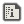# IF StatementcomputableeCreated:

# Description

IF statements are a type of conditional, which means that the code is run differently depending on certain conditions provided. IF statements are among the most important commands in any programming language, because without conditionals, no program would be interactive. Comparators are important in IF statements. They compare two objects. The comparators are as follows:
```== (is equal to)
!= (is not equal to)
> (is greater than)
< (is less than)
>= (is greater than or equal to)
<= (is less than or equal to)
! (invert what follows)
&& (both sides of && must evaluate to TRUE to return TRUE)
|| (either side of || must evaluate to TRUE to return TRUE)
XOR (only one side of XOR can be TRUE to return TRUE)
TRUE (TRUE)
FALSE (FALSE)
```
An IF statement actually contains 5 keywords to learn: IF, THEN, ELSEIF, ELSE, and ENDIF.

## IF

IF initiates an IF chain. After the IF keyword, you provide a comparative statement, meaning, you compare two or more things. If the comparative statement after the IF keyword evaluates to TRUE, then everything after the THEN keyword will be executed. The following are a couple of examples of comparative statements that evaluate to TRUE:
```5 == 5
7 >= 2
"string a" != "string b"
!FALSE
TRUE && TRUE
TRUE || FALSE
TRUE XOR FALSE
```
The following are a couple of examples of comparative statements that evaluate to FALSE:
```5 == 4
7 < 2
"string a" == "string b"
!TRUE
TRUE && FALSE
FALSE || FALSE
TRUE XOR TRUE
```

## THEN

THEN is only executed if the comparative statement after IF evaluates to TRUE. If the comparative statement that follows IF is evaluated to TRUE, all code between THEN and ELSE/ELSEIF/ENDIF will be evaluated. THEN is required in any IF statement.

## ENDIF

ENDIF ends a multi-line IF statement. It concludes the statement, and any code after will not be affected by any conditional statements inside the IF block. ENDIF is required in any multi-line IF statement.

## ELSE

ELSE is only executed if the comparative statement after IF evaluates to FALSE. If the comparative statement that follows IF is evaluated to FALSE, all code between ELSE and ENDIF will be evaluated. ELSE is optional in an IF statement.

## ELSEIF

ELSEIF is short for ELSE IF. ELSEIF contains another comparative statement after it, and extends to another THEN statement and possibly another ELSE statement. If the comparative statement in the previous IF or ELSEIF command evaluated to false, then the comparative statement after ELSEIF is checked. The same rules apply as if it were simply an IF statement. There can be as many ELSEIF statements in a chain as you want.

# Program Example

Example of single-line IF statement syntax.
```IF 5==5 THEN PRINT "This is the reflexive property of equality"

'Output on screen:
'This is the reflexive property of equality
```
Example of multi-line IF statement syntax.
```IF 5==5 THEN
PRINT "Do I really need to repeat what I said in the previous example?"
ENDIF

'Output on screen:
'Do I really need to repeat what I said in the previous example?
```
Example of comparing strings.
```IF "String A"=="String B" THEN
ENDIF

'Code evaluated to FALSE
```
Example of comparing variables.
```VAR NUM1%=5
VAR NUM2%=6
IF NUM1%<NUM2% THEN
ENDIF

'Code evaluated to TRUE (5<6)
```
Example of ELSE.
```IF 5>8 THEN
PRINT "5 is greater than 8"
ELSE
PRINT "5 is not greater than 8"
ENDIF

'Output on screen:
'5 is not greater than 8
```
Example of ELSEIF
```IF 5>5 THEN
PRINT "5>5"
ELSEIF 5==5 THEN
PRINT "5=5"
ELSE
PRINT "5<5"
ENDIF

'Output on screen:
'5=5
```
Example of comparing multiple things in one IF statement.
```IF 5>3 && "apples"!="oranges" THEN PRINT "All good!"

'Output on screen:
'All good!
```If uo